Courses

# Test: Exponents- 1

## 15 Questions MCQ Test Quantitative Aptitude for SSC CGL | Test: Exponents- 1

Description
This mock test of Test: Exponents- 1 for GMAT helps you for every GMAT entrance exam. This contains 15 Multiple Choice Questions for GMAT Test: Exponents- 1 (mcq) to study with solutions a complete question bank. The solved questions answers in this Test: Exponents- 1 quiz give you a good mix of easy questions and tough questions. GMAT students definitely take this Test: Exponents- 1 exercise for a better result in the exam. You can find other Test: Exponents- 1 extra questions, long questions & short questions for GMAT on EduRev as well by searching above.
QUESTION: 1

Solution:
QUESTION: 2

Solution:
QUESTION: 3

### If 22 x 83 = 2 x 4z, what is the value of z?

Solution:

Given 22 x 83 = 2 x 4z

⇒ 22 x 23.3 = 2 x 22z

⇒ 211 = 21+2z

⇒ 11 = 1 + 2z

⇒10 = 2z

⇒ z = 5

QUESTION: 4

Find the value of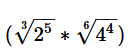?

Solution: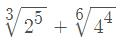⇒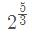+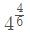⇒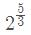⇒+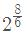⇒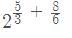⇒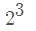⇒ 8

QUESTION: 5

If n is a positive integer greater than 2, what is the greatest prime factor of 3n + 3n + 3n – 3n-2?

Solution:
QUESTION: 6

If ‘x’ and ‘y’ are non-negative integers, what is the value of x + y?

(1) x4 is even, where x is a prime number.

(2) xy is odd.

Solution:

(1) x = 2, y is unknown. Insufficient

(2) x and y unknown. Insufficient

(1)+(2) only possibility x=2, y=0; Sufficient

C is correct

QUESTION: 7

Find the value of n that satisfies the equation 2(-3)4n = 18(27)n+2

Solution:

We need to find the value of n, given 2(−34n)=18(27)n+2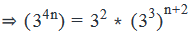(Cancelling 2 on both sides. Also making use of the fact that 32k = (-3)2k)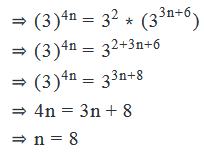QUESTION: 8

The intensity of radiation emanating from a nuclear event is measured on an exponential scale where the second and fourth points indicate intensities of 25 and 625, respectively. On that scale, what would the intensity at the third point be?

Solution:

Step 1: Question statement and Inferences

We are given that the scale is an exponential scale. This means that every term on this scale is equal to the previous term multiplied by a fixed value.

Step 2 & 3: Simplifying the fraction and calculating the final answer

We are given that 2nd term on the scale = 25 = 52

4th term on the scale = 625 = 54

From here, we can discern the pattern that:

The nth term on the scale = 5n

Thus, the 3rd term on the scale = 53 = 125

QUESTION: 9

Positive integer P lies between 1 and 30. What is the value of P?

(1) P has at least two prime factors

(2) The cube of P is less than 300

Solution:

Steps 1 & 2: Understand Question and Draw Inferences

Given that P is a positive integer and that 1 < P < 30.

We need to find the value of P

Step 3: Analyze Statement 1

Given that P has at least two prime factors.

• P is a composite number with two or more prime factors.

However, there are more than one composite number between 1 and 30. The possible values of P are: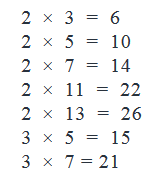Clearly, Statement (1) doesn’t lead us to a unique value of P.

Not Sufficient.

Step 4: Analyze Statement 2

Statement 2 says that the cube of P is less than 300.

Let us list out the cubes of natural numbers.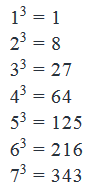There are 5 natural numbers between 1 and 30 whose cubes are less than 300.

Statement 2 alone is not sufficient to arrive at a unique answer.

Step 5: Analyze Both Statements Together (if needed)

From Statement 1,

Possible values of P: 6, 10, 14, 15, 21, 22, 26

From Statement 2,

Possible values of P: 2, 3, 4, 5, 6

By combining the two statements, we get:

P = 6

Therefore statement 1 and statement 2 combined are sufficient to arrive at a unique answer.

QUESTION: 10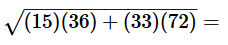Solution:

Given expression is: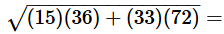Note that 15 = 3*5

36 = 62

33 = 3*11

72 = 36*2 = 62*2

Therefore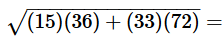QUESTION: 11

The half-life of a radioactive element is defined as the amount of time required for the amount of the element to decrease by half. If the half-life of an element is 3 years, how long will it take for the element to be reduced to one-eighth of its original amount?

Solution:

Let us first understand the given information.

The question states the following about half-life:

• Half-life is defined as the time taken for the mass of a radioactive substance to reduce to half.

The interesting thing to notice here is that the Half-life apparently is not dependent on the initial mass of the radioactive substance.

In other words, if a particular radioactive substance’s half-life is 2 years, then the time taken by it

• To reduce from 1000 kg to 500 kg is 2 years
• To reduce from 500 kg to 250 kg is 2 years
• To reduce from 250 kg to 125 kg is 2 years and so on.

This makes it easier for us to calculate the time taken by the radioactive substance to decay because we will be simply counting the number of half-lives taken.

We need to find the approximate time taken for a X grams block of the radioactive element to reduce to about X/8 grams.

As we noted earlier,

In 1 half-life, the amount of element left = ½ * X

In 2 half-lives, the amount of element left = ½ * ½ * X = (½)2 * X

Let’s say the number of half-lives taken = n.

Then in n half-lives, the amount of element left = (½)n * X

Given that the mass left is 1/8 th of the original amount.

Therefore amount of element left = X/8

This gives us (½)n * X = X/8

• (½)n = 1/8
• (½)n = (½)3
• n = 3

Therefore the time taken = 3 half-lives = 3*3 = 9 years.

QUESTION: 12

if,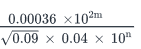= 3 x 10, then 4m - 2n =

Solution:

We need to find the value of 4m – 2n.

Given expression: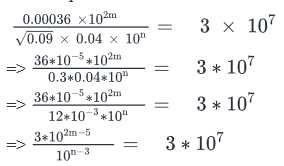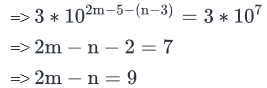Therefore, 4m -2n =2 * (2m -n) =18

QUESTION: 13

Is xy ≥ 1 if both x and y are non-zero integers?

(1) y = -x

(2) y = 2k where k is a non-zero integer

Solution:

Step 1: Question statement and Inferences

xy ≥ 1 if one of two things is true. This is when

1. x and y are both positive (23), or
2. x is negative and y is positive and even

Note, a negative y does not helps us since any integer raised to a negative power cannot be greater than 1. Hence lets figure out if x and y fulfill one of the two criteria above.

Step 3: Analyze Statement 1

1) y = -x

Statement 1 says that y and x are of opposite signs.

Let’s say that x = 2

This means, y = -2

So xy = 2-2 = ¼ which is less than 1

Let’s now consider the case x = -2

This means, y = 2

So, xy = (-2)2 = 4, which is greater than 1

Thus, we realize that Statement 1 does not lead us to a definitive answer. So, Statement 1 is NOT SUFFICIENT

Step 4: Analyze Statement 2

y = 2k where k is a non-zero integer

We are given that k cannot be equal to zero.

Can k be a negative integer?

The answer is No. Because if k were to be a negative integer, then y would become a fraction (For example, say k = -3. Then y =2-3 = 1/8)

But, we are given that y is an integer.

This is only possible if k is a positive integer.

Thus, k can have only positive values and y is a positive multiple of 2.

x may be a positive or a negative integer, but when it is raised to an even power, the result is always going to be positive.

If x = -1 or 1, then the value of xy will be equal to 1. For any other allowed value of x (remember: x = 0 is not allowed), the value of xy will be greater than 1.

Statement 2 is SUFFICIENT

QUESTION: 14

Find the value of positive integer P that lies between 1 and 30 and is a perfect square.

(1)  P has at least one Prime factor

(2)  The cube of P is less than 300

Solution:

Steps 1 & 2: Understand Question and Draw Inferences

Given that P is positive and 1 < P < 30.

It is also given that P is a perfect square.

This means, that P is the square of an integer.

Therefore the possible values of P are 4, 9, 16, and 25.

Step 3: Analyze Statement 1

Given that P has at least one prime factor.

EVERY number greater than 1 has at least one prime factor.

This statement doesn’t help us to eliminate any value out of the four possible values of P.

So statement 1 is not sufficient to arrive at a unique answer.

Step 4: Analyze Statement 2

Given that cube of P is less than 300.

Observe that only 4 satisfies this condition.

43=64

93=729

(Since 93 itself is greater than 300, it is clear that 163 and 25will also be greater than 300.)

Therefore the only possible value for P is 4.

Statement 2 is sufficient to arrive at a unique answer.

Step 5: Analyze Both Statements Together (if needed)

We arrived at a unique answer in step 4. Hence this step is not required.

QUESTION: 15

If k is an integer then find the value of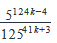(1) 2r – 2k = 26, where r is an integer

(2) 14 + k = 3k – 2r, where r is an integer

Solution:

Given that k is an integer, we need to find the value of the expressionSimplifying this expression, we get: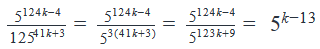For this we need the value of k.

Step 3: Analyze Statement 1

Given 2r – 2k = 26 (This also tells us that r≠k and r > k)

• (2k)*(2r-k – 1) = 26………… (I)

Let us try to understand the equation in (I)

The RHS is purely a power of 2.

So there cannot be a power of any other prime in the product on the LHS.

2k is definitely a power of 2.

But what about (2r-k – 1)?

It is evident that it is an odd number. (2 raised to the power any positive number will be an even number. Even number – Odd number = Odd number)

Therefore the only possible value for (2r-k – 1) is 1. (Any other odd number would introduce a new prime in the LHS.)

• 2r-k – 1 = 1

If 2r-k – 1 = 1 then from (I), 2k = 26

• k = 6

We got a unique value of k. So we can calculate the value of the given expression.

Statement 1 is sufficient to arrive at a unique answer.

Step 4: Analyze Statement 2

Given equation is

14 + k = 3k – 2r

• 2k = 2r + 14
• k = r + 7

This doesn’t help us calculate the value of the given expression.

Statement 2 alone is not sufficient to arrive at a unique answer.

Step 5: Analyze Both Statements Together (if needed)

We arrived at a unique answer in step 3. Hence this step is not needed.